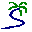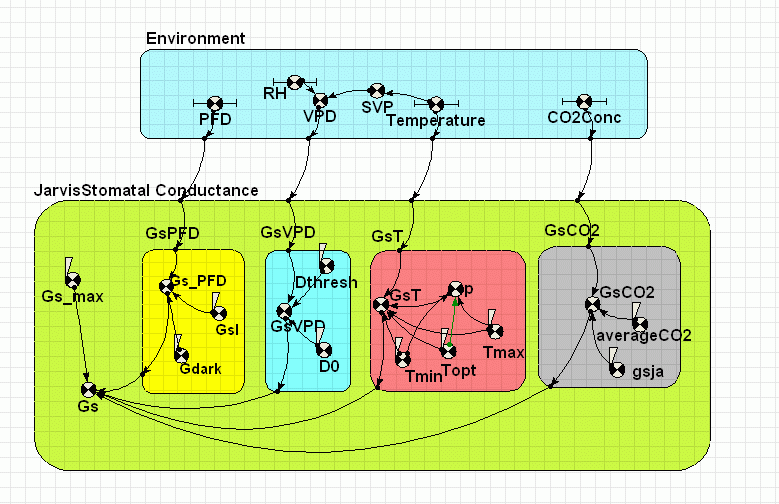# Model entry

Stomatal conductance by law of limiting functions after Jarvis - Model catalogue - Simulistics.com

 Search Simulistics Model catalogue Listed by keyword Listed by ID Listed by title Listed by date added

# Stomatal conductance by law of limiting functions after Jarvis

Model : JarvisStomatalConductance
Simile version : 4.0
Keywords : Stomatal conductance ; Ecophysiology ; Evapotranspiration ; Law of limiting factors ;

## Description

An empirical model of conductance of water vapour etc. through stomata (leaf pores). The predicted conductance is the product of a maximum conducatance and a number of normalized functions. The functions are response curves to particular environmental variables. In this model the responses are to: photon flux density (PFD), vapour pressure deficit (VPD), leaf temperature and CO2 concentration.

To run the model download the following files to the same directory. Met.csv is required by the parameter file params.spf

## Files

### Model file

Click on the icon to download the model file. (You will need Simile to examine and run the model. A free evaluation version is available from the products page.)

Some browsers may attempt to display the model file, rather than open it in Simile; in this case, use the browser back button to return to this page, and use the context menu (invoked by right-clicking on the link) to save the target file to disk.TestJarvisStomataModel.smlMet.csvparams.spfDisplays.shf

## Diagram## Equations

```
Equations in JarvisStomatal Conductance

Variable   Gs : Stomatal conductance to water vapour Jarvis model
Gs = Gs_max*Gs_PFD*GsVPD*GsT*GsCO2
Where:
Gs_PFD=GsPFD/Gs_PFD
GsVPD=GsVPD/GsVPD
GsT=GsT/GsT
GsCO2=GsCO2/GsCO2
No comment

Equations in Environment

Variable   SVP : Saturated Vapour Pressure
SVP = 0.611*exp(17.27*Temperature/(Temperature+237.15))
temperature is in degrees C  svp is the saturated vapour pressure,in kPa from the                                   Tetens' formula from Montieth and Unsworth, 1990, Principles of     Environmental Physics p10, adapted for T in deg C                  Values are within 1 Pa upto 35 deg C

Variable   VPD
VPD = SVP*(1-RH)

Equations in GsPFD

Variable   Gs_PFD
Gs_PFD = Gsl*(PFD+Gdark/Gsl)/(1+Gsl*(PFD+Gdark/Gsl))
Where:
PFD=../../Environment/PFD
No comment

Equations in GsVPD

Variable   GsVPD
GsVPD = g=1-(VPD-Dthresh)/(D0-Dthresh),max(min(g,1.0),0)
Where:
VPD=../../Environment/VPD

Equations in GsT

Variable   GsT
GsT = g=(Temperature-Tmin)*(Tmax-Temperature)^p/((T0-Tmin)*(Tmax-T0)^p),min(max(g,0),1)
Where:
Temperature=../../Environment/Temperature
T0=Topt

Variable   p
p = (Tmax-Topt)/(Topt-Tmin)

Equations in GsCO2

Variable   GsCO2
GsCO2 = g=1-gsja*(CO2Conc-averageCO2),max(min(g,1.0),0.0)
Where:
CO2Conc=../../Environment/CO2Conc

```

## Results

||